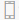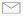•• 9958331191
•• mybook@vishvbook.com
• Shopping Cart

## Math Text Books

Excel in Mathematics is a series of 8 books for Classes 1 through 8. The books comply with the latest NCERT syllabus.

#### Excel in Mathematics - 1

Excel in Mathematics is a series of 8 books for Classes 1 to 8. The books comply with the latest NCE..

INR234.00

#### Excel in Mathematics - 2

Excel in Mathematics is a series of 8 books for Classes 1 to 8. The books comply with the latest NCE..

INR243.00

#### Excel in Mathematics - 3

Excel in Mathematics is a series of 8 books for Classes 1 to 8. The books comply with the latest NCE..

INR252.00

#### Excel in Mathematics - 4

Excel in Mathematics is a series of 8 books for Classes 1 to 8. The books comply with the latest NCE..

INR270.00

#### Excel in Mathematics - 5

Excel in Mathematics is a series of 8 books for Classes 1 to 8. The books comply with the latest NCE..

INR279.00

#### Excel in Mathematics - 6

Excel in Mathematics is a series of 8 books for Classes 1 to 8. The books comply with the latest NCE..

INR293.00

#### Excel in Mathematics - 7

Excel in Mathematics is a series of 8 books for Classes 1 to 8. The books comply with the latest NCE..

INR297.00

#### Excel in Mathematics - 8

Excel in Mathematics is a series of 8 books for Classes 1 to 8. The books comply with the latest NCE..

INR311.00

Showing 1 to 8 of 8 (1 Pages)